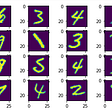# How do ML Models actually do Gradient Descent?

## Get an Intuition for the Difference between SGD vs RMSprop vs Adam optimizers

When people first start out machine learning in PyTorch, you might see a PyTorch script like this.

You instantiate an optimizer, obtain your labels for the data, calculate your loss, and backpropagate to minimize your loss function.

The most important line in all of this code (the first one), however, is obscured by all of the high-level abstract code that PyTorch offers, and though this makes coding neural networks easy in the long run, it prevents us from getting an intuition about which optimizers work better and what the differences are between the different types of optimizers.

In this article, I’ll simply explain the three most common kinds of optimizers, show how the optimizers differ and performance, and show you how you can code the optimizers from scratch.

1. A quick refresher on gradient descent (SGD)
2. SGD with momentum
3. RMSprop

For all of our examples, we’ll be looking at either simple linear regression or MNIST digit classification

We’ll start off with a quick refresher on gradient descent — feel free to skip if you are familiar.

The explanation for this section comes from the SGD notebook that is part of lesson two of the fast.ai v3 course on Kaggle (search notebook name: fastai-v3-lesson-2-sgd)

Given this scatterplot, our goal is to have the machine learning model to recover the two parameters of our regression model: m and b.

The first thing that we have to do is define a loss function. This loss function allows us to determine how accurate our model’s predictions are → Therefore, we can make changes to the model’s parameters based on the error values.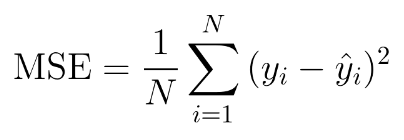Mean squared error loss captures how close our predicted y value (y “hat”) is to the actual “y”

Now how do we actually make these changes to our loss values? Through gradient descent.

The gradient is the derivative of the error with respect to the parameter in question. Let’s break that down.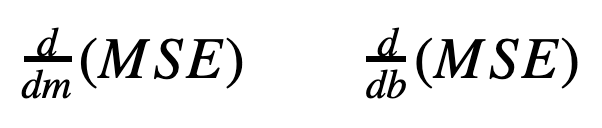Left: Gradient of the MSE error with respect to m (our slope). Right: Gradient of the MSE error with respect to b (our intercept)

Essentially, we’re trying to measure how much our our error changes with an infinitesimal increase in the parameter of interest. If the MSE goes up, then the derivative is positive. That means we should decrease the parameter. If the gradient is negative, that means that increasing the parameter will decrease the error, so we increase the parameter.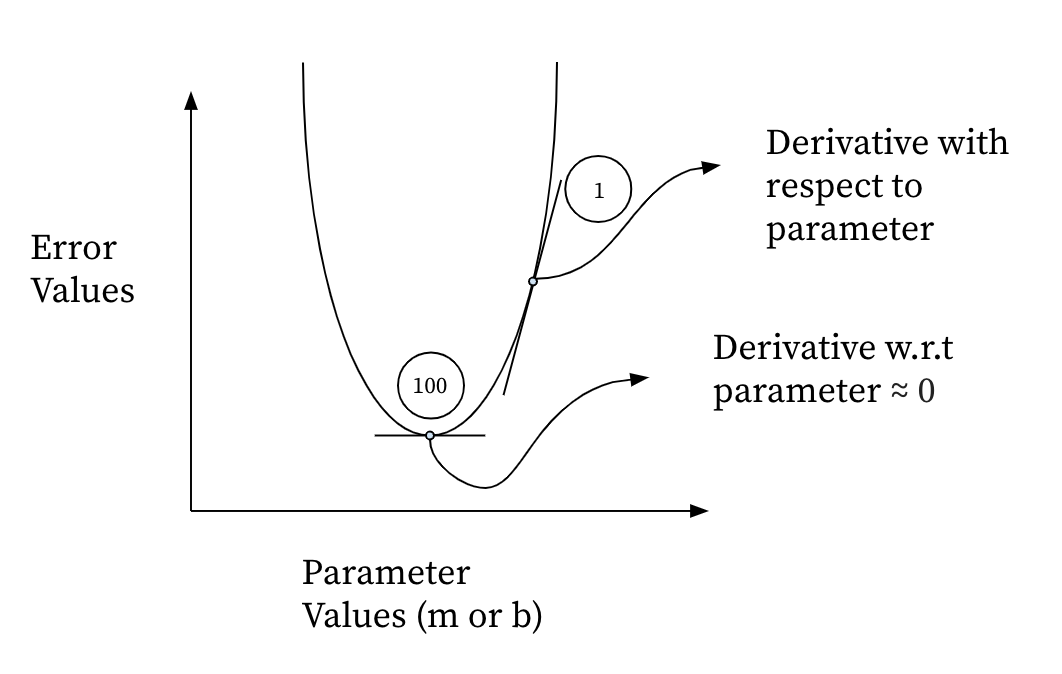Each of the parameters that we defined above (m and b) have a graph like this which shows how the value of parameter varies with the value of the error. The way that we measure whether we’re at the minimum error value is whether our derivate with respect to the parameter is approximately 0.

Now our goal is to get a gradient of zero — that is, by increasing our parameter infinitesimally, the error does not change.

That’s it! That’s gradient descent. Let’s take everything that we discussed and put it into a small concise, generalizable formula (to situations beyond just linear regression).We update the parameter theta-j by subtracting a learning rate value multiplied by the gradient of the error with respect to the parameter.

Now let’s try implementing this in a gradient descent excel spreadsheet and see how well this works! To work along with me, retrieve the gradient descent spreadsheet from this link: https://course.fast.ai/videos/?lesson=5.

Our new parameters are m = 30 and b = 2.

Let’s run through this data for 4 epochs. An epoch is defined by the time period after which our model has observed all of our data points.

Something’s wrong.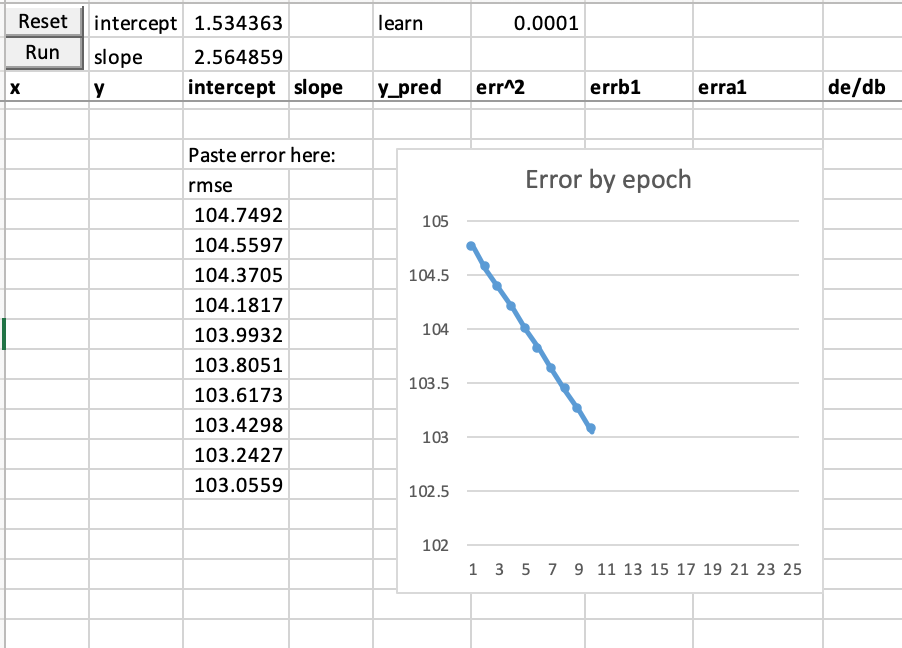Our error has gone down but very slightly. Look at our slope and intercept — they’re nowhere close to where they need to be!

How about increasing the learning rate alpha? You’d think that that would work, but it results in errors in the spreadsheet due to what’s known as the exploding gradient problem — the gradients become too large, and suddenly we’re reaching error values of 10¹⁵³.

## Momentum

Let’s try SGD with something called momentum. Momentum tells us to calculate the step (the value that we subtract from the parameters) slightly differently. Instead of subtracting the derivative with respect to the parameter times the learning rate, we’ll subtract (0.9 *the previous step) + (0.1 * the gradient).

Essentially, we’re telling our model give only 10% importance to the gradient that we just calculated and 90% importance to the the previous step that the model made to the parameters.

Think of it like inertia, if the parameter decreased a ton in the last step, it’ll keep decreasing due to the momentum that it has acquired — the new gradient might slow it down, but only a little bit. This can help us to converge on the global minimum much faster.

We’ll name our new parameter momentum as β.

Now our new step looks something like…

Here’s the spreadsheet after two epochs with momentum. In the top left, you can see that our results aren’t even better than the last spreadsheet… Onto our next edition — RMSprop.

## RMSprop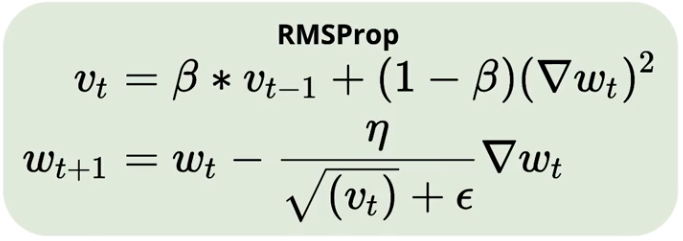v(t) is the exponentially weighted moving average of the weights. The step is calculated by taking the gradient times the learning rate and dividing by the square root of the exponentially weighted moving average v(t).

V(t) is what’s called the exponentially weighted moving average. The reason it’s called exponentially weighted is because the (1-beta) * gradient squared factor allows it to keep a track record of all of the previous squared gradient values.

Notice that v(t) is in the denominator of the step function. This creates an inverse relationship, where if the exponentially weighted moving average is larger, then the step is smaller, and if the average is smaller the step is larger.

Remember when our parameters where getting updated super slowly in the first SGD spreadsheet? Now, with RMSprop, our parameters will be updated much faster due to the inverse relationship between gradient and step size.

Look at that! With 2 epochs, we get to a slope of 4.72 — not quite to the slope of 30 that we’re aiming for, but three times better than the slopes of 1.5 that we got through SGD.

There’s one more improvement coming along…

Now what we’re going to do is combine the ideas of momentum with the RMSprop principle involving the inverse relationship.

Here’s a surprise — adam is actually nothing new. Think of it as a combination between the momentum and RMSprop implementation.

To simplify things for yourself, first ignore the m(t) on the top — notice the inverse relationship preserved with v(t). As the exponentially weighted moving average of the gradients decreases, the step increases in order to converge faster. Now ignore the v(t) and look at m(t). This is a direct relationship — if the previous step is large, then mt will also be large, increasing the value of the step since it’s in the numerator.

There we have it — a small v(t) and a large (m(t)) will allow for changes in our parameters that occur much more than fast than any other optimizers we’ve looked at so far.

We’re at 2 epochs and…

b=29.25! — that’s very very close to our goal of m=30.

a has slightly overshot but is also approximately close to 2.

# Code implementations and loss plots in PyTorch for MNIST data set

Replicating these implementations of the optimizers that I’ve coded from scratch should help you gain a more solidified understanding of the math involved.

Here are the three code implementations for momentum, RMSprop, and Adam respectively placed side by side. Notice the weight decay element involved in all of the code examples. Weight decay can also be called L2 regularization. Penalizing our loss function for large weight values allows us to generalize better to an imaginary test set.

First, let’s familiarize ourselves with the model — here we’re using a simple feedforward network with a single linear layer inherited from the nn.Linear module. There are two parameters. The first is a weight matrix of dimensions 784 by 10. The second is a bias vector of dimensions 1 by 10. Our goal is to test out the different optimizers to see which one can minimize the loss in the shortest interval of time (our loss function is MSE loss)Notice how there are two values stored for each initialized parameter

Before coding the optimizers, I initialized three parameters that are involved in optimization. The first is prev_step — this is the parameter that is multiplied by the momentum constant beta during SGD with momentum. Next, we have prev_mo_step — this is used to calculate to our new m(t) value for the adam optimizer. And finally, we have prev_exp_avg_step — this is the value of the stored previous exponentially weighted moving average of the squared gradients (represented by v(t)).

## SGD

Here’s the code for SGD. First, we calculate the predicted y values and implement weight decay where we add the same of the squared gradients to our w2 variable. We then use loss.backward to calculate the gradients (p.grad).

After setting torch.no_grad()==true, we then update our parameters p.

The calculation of step[i] is the most important line in this code. Notice how we multiple p.grad by (1-momentum) and multiply the previous step by the momentum.

After we calculate the step, we subtract the learning rate multiplied by the step with the .sub_() operator.

## RMSprop

Finally, after resetting the gradient to zero, we set the previous step equal to our the step that we just executed on to prepare ourselves for the next update.

The only thing that differs between the code for each of these optimizers is the input arguments and the way that step[i] is calculated.

In RMSprop, our step is calculated in two steps. First we must get the exponentially weighted moving average v(t). As seen in the code, we square our gradient multiply it by (1-momentum) and then take our previous weighted average and multiply by the momentum.

To calculate the step, we take the gradient and divide by the root of this newly calculated v(t), and finally, we update our parameters. Similar to SGD, we must set a variable initializing our previous step (prev_exp_avg_step) before returning loss values.

Again — no difference except for how the step is calculated, except this time we add one more parameter mo_step. The step[i] values for adam are calculated similarly to RMSprop — we still divide by the root of the exp_avg_step. However, this time, the dividend is our mo_step[i]. Here you can clearly see how adam takes into account both our m(t) and v(t).

The momentum1 and 2 arguments are used to set the coefficients for the linear combinations used to constitute the momentum and exp_avg steps (I used 0.9 for both in this case).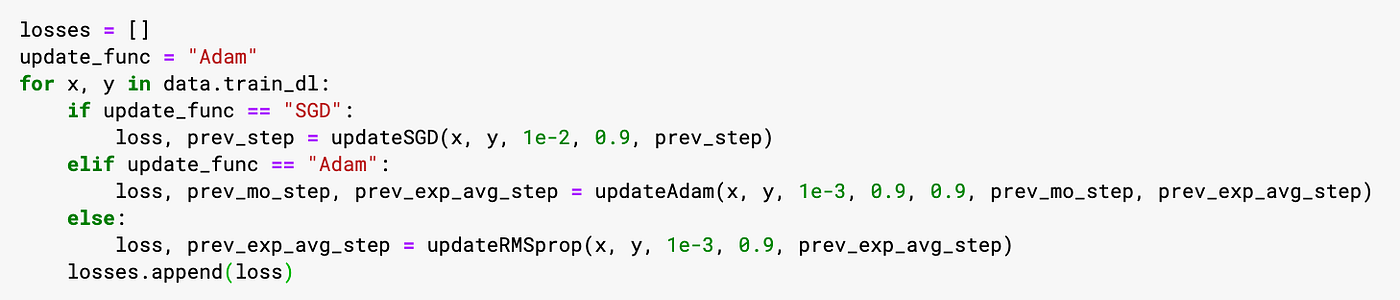Pytorch script passing input into each of the optimizers and appending loss to a list

Here we acquire data and labels from the dataloader and pass arguments into our optimizers. Notice how for each optimizer we also retrieve previous step values as returned values, so that we can pass these in as arguments for our next update.

## Loss Plots

Here’s an exercise — try to guess which optimizer each of the loss plots below belongs to, using your understanding of the rate at which each optimizer does descent.

Notice how the loss plot for SGD doesn’t really converge at an optimum until ~800 updates. Adam and RMSprop converge at around the same time (~250 updates) but notice how Adam’s descent is slightly steeper.

## Why this matters

There are many nuances involved in the performance of deep learning models, from the number of hidden to layers to optimizer used to learning rate set. Most beginners might not consider it important to learn about optimizing these initially, but the faster you learn these nuances — the faster you’ll surprise yourself with state of the art results.

Keep an eye out on soon for an article on optimizing learning rates 👀.

Shoutout to Jeremy Howard, founder of the fast.ai library and course, on which much of this article is based. I’m super grateful for your drive to make state-of-the-art deep learning results accessible to everyone.

Hey! I’m Mukundh Murthy, a 16 year old passionate about the intersection between machine learning and drug discovery. Thanks for reading this article! I hope you found it helpful :)

Feel free to check out my other articles on Medium and connect with me on LinkedIn!

If you’d like to discuss any of the topics above, I’d love to get in touch with you!(Send me an email at mukundh.murthy@icloud.com or message me on LinkedIn) Also, feel free to check out my website at mukundhmurthy.com.

--

--

--

## More from The Startup

Get smarter at building your thing. Follow to join The Startup’s +8 million monthly readers & +756K followers.

## Geospatial Natural Language Processing## Handling Massive Data Rates with Localized Sketching## Major Updates to the Most Popular Data Science Frameworks in 2019## Humming with the bot## 5 Challenges in Machine Learning that you’ll sure shot run into.## ML Classification algorithms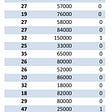## [Deep Learning] Hand gesture recognition## Epidemic Intelligence, part 2: data, models and machine learning in the age of Coronavirus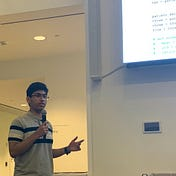## Mukundh Murthy

Innovator passionate about the intersection between structural biology, machine learning, and chemiinformatics. Currently @ 99andbeyond.

## Lights on - PyTorch## Model Pruning in Deep Neural Networks Using the TensorFlow API## CNN: an eye for AI## Solving GANs: NN architecture & feedback